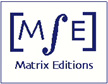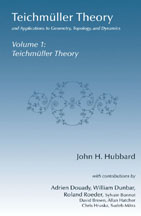Matrix Editions
serious mathematics,
written with the reader in mind

# Preface

### Teichmüller Theory and Applications to Geometry, Topology, and Dynamics Volume 1: Teichmüller TheoryJohn H. Hubbard
ISBN 9780971576629
459 pages, hardcover
smythe-sewn binding

2006, \$77

### Preface and prerequisites

Between 1970 and 1980, William Thurston astonished the mathematical world by an­nouncing the four theorems discussed in this book:

1. The classification of homeomorphisms of surfaces.
2. The topological characterization of rational maps.
3. The hyperbolization theorem for 3-manifolds that fiber over the circle.
4. The hyperbolization theorem for Haken 3-manifolds.

Not only are the theorems of extraordinary beauty in themselves, but the methods of proof Thurston introduced were so novel and displayed such amazing geometric insight that to this day they have barely entered the accepted methods of mathematicians in the field.

The results sound more or less unrelated, but they are linked by a common thread: each one goes from topology to geometry. Each says that either a topological problem has a natural geometry, or there is an understandable obstruction.

The proofs are closely related: you use the topology to set up an analytic mapping from a Teichmüller space to itself; the geometry arises from a fixed point of this mapping. Thurston proceeds to show that if there is no fixed point, then some system of simple closed curves is an obstruction to finding a solution.

Thus the proofs of the theorems are somehow similar, although the details and difficulty are very different. In particular, a collection of preliminary results about Teichmüller spaces is required for all four.

These theorems have been quite difficult to approach. Part of the reason is that Thurston never published complete proofs of any of them.

Other people did: Fathi, Laudenbach and Poenaru published proofs of his results on surface homeomorphisms, Douady and Hubbard published a proof of the topological characterization of rational functions, and Otal published proofs of both hyperbolization theorems. Still, a gap remained: in order to read these papers, a reader must have a very broad background, since the complex analysis is often quite foreign to topologists and geometers, and the topology and geometry are quite as foreign to analysts.

In this book, I propose to gather all the necessary material and to provide complete proofs with a minimum of prerequisites -- assuming the results of a pretty solid first year of graduate studies, but very little beyond.

The book is divided into two volumes. The first sets up the Teichmüller theory necessary for discussing Thurston's theorems; the second proves Thurston's theorems, providing more background material where necessary, in particular for the two hyperbolization theorems.

### Introduction to volume 1

In the first volume I have given quite a complete treatment of Teichmüller theory. Of course I have collected the material that will be needed in the second volume, but I have also proved a number of other results that struck me as interesting in themselves and in the spirit of the book, such as the universal property of Teichmüller spaces, Royden's theorem on automorphisms of Teichmüller spaces, and Wolpert's theorem on the symplectic structure of Teichmüller spaces. The treatment is considerably more ``topologist friendly'' than most other books on the subject, almost all written by analysts with a different outlook on the subject than that required by Thurston's needs.

Highlights of volume 1 are:

the uniformization theorem - the grand-daddy of all hyperbolic geometry

the collar theorem - providing a caricature of all Riemann surfaces, which makes it possible to grasp all of them simultaneously. The proof given here is quite different from the standard.
the mapping theorem - the essential tool from complex analysis, providing the flexibility to construct all the objects under discussion

the Douady-Earle extension theorem - useful throughout complex analysis; for us, its main purpose will be showing that all Teichmüller spaces are contractible

the Slodkowski's theorem - essential for understanding the Kobayashi metric of Teichmüller spaces. The proof given here is quite different from the standard.

Teichmüller's theorem on extremal mappings - essential for understanding the Kobayashi metric of Teichmüller spaces.
the construction of Teichmüller space and its tangent and cotangent spaces Mumford's compactness theorem

Each of these results is important in its own right, and has many applications beyond Thurston's theorems.

Besides the core results above, I have taken a number of scenic detours: hyperbolic trigonometry, curvature of conformal metrics, the 1/d-metric on plane domains (one of Thurston's favorites), fundamental domains of arithmetic groups (the proof of the Poincaré polygon theorem may be a real improvement on earlier presentations), trouser decompositions of arbitrary hyperbolic surfaces, quasi-Fuchsian reciprocity, the construction of Fenchel-Nielsen coordinates, Royden's theorem on analytic automorphisms of Teichmüller spaces, and Wolpert's theorem on the symplectic structure of Teichmüller space.

The appendices contain a number of topics that although more or less standard are not often covered in first-year graduate courses. The last four really represent an alternative ``sheaf-theoretic'' approach to Teichmüller theory, at least for Riemann surfaces of finite type. This approach is well-adapted to the study of moduli problems in higher dimensions, but is not so well adapted to Thurston's theorems.

### Why another book on Teichmüller theory?

Since the publication in 1966 of Lectures on quasiconformal mappings by Lars Ahlfors, a number of books on Teichmüller theory have appeared, including The Real Analytic Theory of Teichmüller Space by W. Abikoff (1980), The Complex Analytic Theory of Teichmüller Spaces by S. Nag (1988), An Introduction to Teichmüller Spaces by Y. Imayoshi and M. Taniguchi (1992), and Quasiconformal Teichmüller Theory by F. Gardiner and N. Lakic (1999).

These books are all excellent, and I recommend them highly. I have used all of them at various places when writing the present book. But they all have a somewhat different focus than the present one, more analytic and less topological, and they don't quite contain the prerequisites for Thurston's theorems. They are less self-contained, and do not hesitate to refer to the literature; these references are sometimes quite difficult to read. Someone (a topologist or geometer, perhaps) wanting to learn Thurston's theorems might well find them daunting. My main justification for writing the present book is to make that task easier.

### Introduction to volume 2

Note: This "volume 2" has grown to volumes 2, 3, and 4.

A detailed introduction to volume 2 will appear there; for the present, let the following suffice. For the classification of homeomorphisms of surfaces and the topological characterization of rational functions, volume 1 gives adequate background, and these theorems are covered in Chapters 8 and 9. The hyperbolization theorems are far more elaborate, and require quite a few further results.

Chapter 10 presents background on the geometry of hyperbolic space and on Kleinian groups, which is essential for all subsequent material. Chapter 11 covers various rigidity results: the Ahlfors finiteness theorem, the McMullen rigidity theorem, and Mostow's rigidity theorem, which is a special case. Chapter 12 proves the hyperbolization theorem for 3-manifolds that fiber over the circle.

The hyperbolization theorem for Haken manifolds requires further background material. Chapter 13 covers the rather extensive 3-dimensional topology that is needed (Dehn's lemma, the loop theorem, hierarchies). Chapter 14 contains a proof of Andreev's theorem. Chapter 15 finally presents the hyperbolization theorem.

The names given here merely hint at the wealth of mathematics on which Thurston was able to draw - and needed to draw - for his own theorems. While writing this book I have had the impression of being engulfed in a grand mathematical symphony, with many famous -and some undeservedly neglected - mathematicians of the past and present coming forth at just the right moment with the result needed for the symphony to continue to its grand finale.

### Prerequisites

I have tried very hard to make this book accessible to a second-year graduate student: I am assuming the results of a pretty solid first year of graduate studies, but very little beyond, and I have included appendices with proofs of anything not ordinarily in such courses. I never refer to the literature for some difficult but important result. Such references are the bane of readers, who often find the slight differences of assumptions and incompatible notations an insurmountable obstacle.

Of course, one can read many parts of the book with much less background, and some material can presumably be picked up ``by osmosis.''

More specifically, courses covering the following topics should be adequate:

#### Real analysis

Ascoli's theorem, Lebesgue integration, classical Banach spaces, distributions and distributional derivatives (especially in Chapter 3). The ergodic theorem is used in Chapter 10 of volume 2, but is proved in an appendix.

#### Complex analysis

Normal families, Montel's theorem, Picard's theorem, subharmonic functions, the area theorem, the Koebe 1/4-theorem (there is a new proof in Chapter 4), Riemann mapping theorem, the definition of Riemann surfaces.

#### Algebra

I assume basic group theory, ring theory, and field theory. A lot of the book is concerned with infinite groups, but I think no serious theorem about them is used. In Chapter 10 of volume 2, we use the Nullstellensatz, but prove it in an appendix of that chapter.

#### Differential manifolds and differential forms

These are used throughout, including Stokes's theorem and the exterior derivative, orientation, Sard's theorem, partitions of unity, and Riemannian manifolds. We also require the Gauss-Bonnet theorem for surfaces (hence curvature for surfaces, geodesics, and geodesic curvature of curves) and the Hopf index theorem, though these are reviewed pretty thoroughly in the text.

#### Topology

We certainly assume that covering space theory and the relation with the fundamental group are well understood. Elementary homology and cohomologytheory, including the Mayer-Vietoris exact sequence, are used in Chapters 1 and 5, and considerably more is used in Chapter 12 in volume 2. But the delicate results of 3-dimensional manifold theory, such as Dehn's lemma, the loop theorem and the existence of hierarchies in Haken 3-manifolds, are all proved in detail. Algebraic Topology by Allen Hatcher is an excellent reference.

I also expect readers to be comfortable with the language of categories and functors, including universal properties and representable functors. I only use the language, no results.